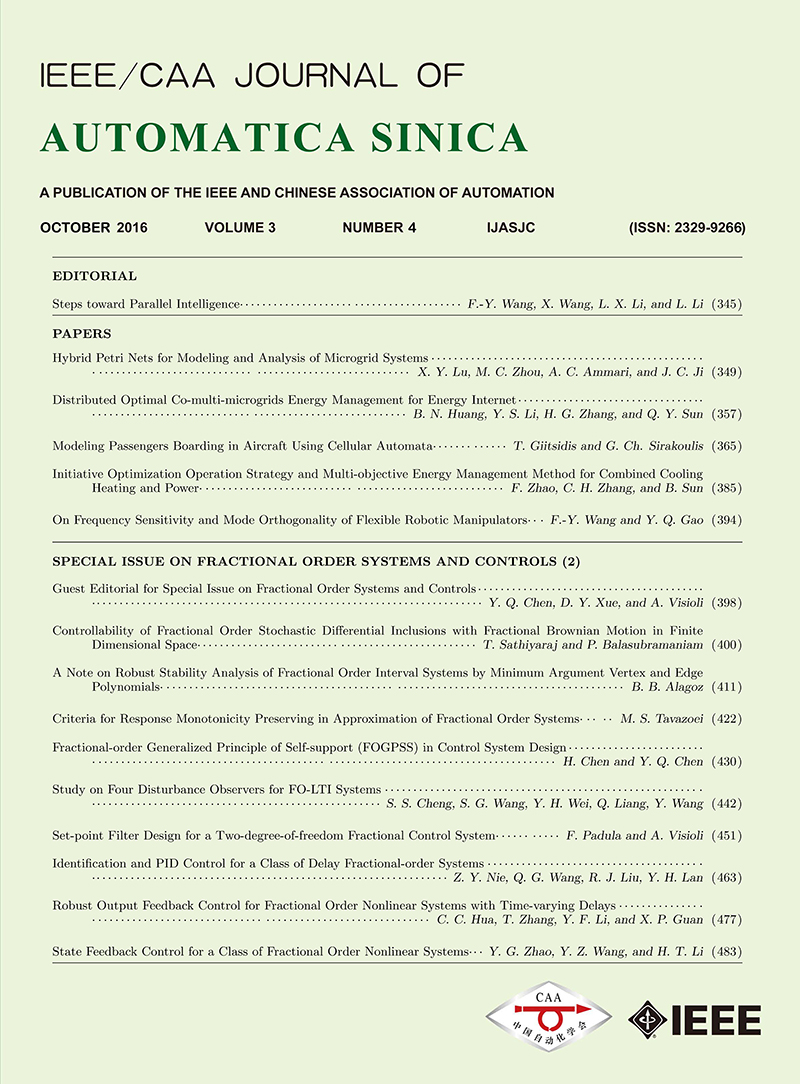A journal of IEEE and CAA , publishes high-quality papers in English on original theoretical/experimental research and development in all areas of automationVolume 3 Issue 4
Oct.  2016

IEEE/CAA Journal of Automatica Sinica

• JCR Impact Factor: 6.171, Top 11% (SCI Q1)
CiteScore: 11.2, Top 5% (Q1)
Google Scholar h5-index: 51， TOP 8
Turn off MathJax
Article Contents
Baris Baykant Alagoz, "A Note on Robust Stability Analysis of Fractional Order Interval Systems by Minimum Argument Vertex and Edge Polynomials," IEEE/CAA J. Autom. Sinica, vol. 3, no. 4, pp. 411-421, Oct. 2016.
 Citation: Baris Baykant Alagoz, "A Note on Robust Stability Analysis of Fractional Order Interval Systems by Minimum Argument Vertex and Edge Polynomials," IEEE/CAA J. Autom. Sinica, vol. 3, no. 4, pp. 411-421, Oct. 2016.# A Note on Robust Stability Analysis of Fractional Order Interval Systems by Minimum Argument Vertex and Edge Polynomials• By using power mapping (s=vm), stability analysis of fractional order polynomials was simplified to the stability analysis of expanded degree integer order polynomials in the first Riemann sheet. However, more investigation is needed for revealing properties of power mapping and demonstration of conformity of Hurwitz stability under power mapping of fractional order characteristic polynomials. Contributions of this study have two folds:Firstly, this paper demonstrates conservation of root argument and magnitude relations under power mapping of characteristic polynomials and thus substantiates validity of Hurwitz stability under power mapping of fractional order characteristic polynomials. This also ensures implications of edge theorem for fractional order interval systems. Secondly, in control engineering point of view, numerical robust stability analysis approaches based on the consideration of minimum argument roots of edge and vertex polynomials are presented. For the computer-aided design of fractional order interval control systems, the minimum argument root principle is applied for a finite set of edge and vertex polynomials, which are sampled from parametric uncertainty box. Several illustrative examples are presented to discuss effectiveness of these approaches.

•Figures(10)  / Tables(2)

## Article MetricsDownLoad:  Full-Size Img  PowerPoint